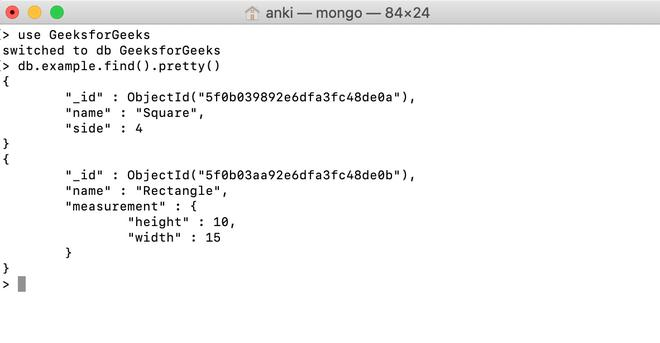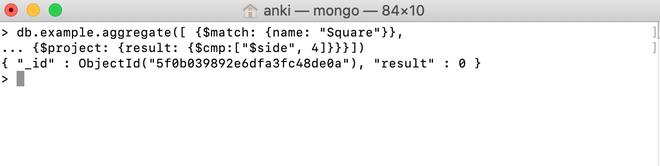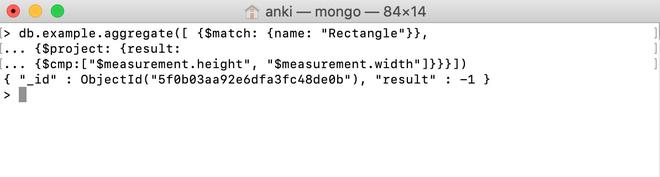Skip to content
Related Articles
MongoDB \$cmp Operator
• Last Updated : 01 Aug, 2020

MongoDB provides different types of comparison expression operators that are used in the aggregation pipeline stages \$cmp operator is one of them. This operator is used to perform a comparison between two values and return the following result according to the condition:

• If the first value is greater than the second value, then this operator will return 1
• If the first value is less than the second value, then this operator will return -1
• If both the values are equal, then this operator will return 0.

Syntax:

```{ \$cmp: [ <expression1>, <expression2> ] }

```

Examples:

In the following examples, we are working with:

Database: GeeksforGeeks

Collection: example

Document: two documents that contain the details of the shapes in the form of field-value pairs.Using \$cmp Operator:

In this example, we are comparing the value of the side field with 4 and \$cmp operator return 0 which means both values are equal.

```db.example.aggregate([{\$match: {name: "Square"}},
... {\$project: {result: {\$cmp:["\$side", 4]}}}])```Using \$cmp Operator in the Embedded Document:

In this example, we are comparing the value of the measurement.height field with the value of the measurement.width field and \$cmp operator return -1 which means both values of measurement.height field is less than the value of the measurement.width field.

```db.example.aggregate([{\$match: {name: "Rectangle"}},
... {\$project: {result:
... {\$cmp:["\$measurement.height", "\$measurement.width"]}}}])```My Personal Notes arrow_drop_up